# Slot machine project using R programming

The slot machine is a game, and it comprises two steps: Generating three symbols randomly from 7 symbols and For the generated three symbols, computing score.

### Algorithm:

• The symbols used in the slot machine are:
• Diamond
• Seven
• 3Bars
• 2Bars
• 1Bar
• cherry
• Zero
• We can take any symbol. It may depend on the choice of the individual.
• From the above symbols, three symbols are generated randomly.
• We can get random symbols using the sample() function in R programming.
• According to the symbols, some prize money will be there.
• Allocation of prize money to the generated symbols also depends on the individual.
```So, for easy understanding let us consider the below amount
of money as prize for this project.
Diamond Diamond Diamond -------------------> 1000
Seven Seven Seven --------------------> 800
3Bars 3Bars 3Bars ------------------> 600
2Bars 2Bars 2Bars --------------------> 400
1Bar 1Bar 1Bar ---------------------> 250
Cherry Cherry Cherry ----------------------> 100

If three symbols are generated with the combination of all
types of bars prize money will be 50 .
If three symbols are generated with the combination of two
Cs and remaining one empty or 0 prize money will be 50.
0 Cherry Cherry------------> 50
Cherry 0 Cherry ------------> 50
Cherry Cherry 0 -------------> 50```
• If the 3 symbols are generated with the combination of one Cherry and the remaining two are empty or 0 prize money will be 20.
• If the three symbols are zeros prize money will be obviously 0.
• If we get Seven-Seven-Diamond, Diamond will be considered as Seven. If Diamond presents the prize money will be doubled so, the prize money will be 800 * 2 = 1600.
• If we get Seven-Diamond-Diamond, here Diamond occurred twice so, the prize money will be doubled twice. The prize money will be ( 800 * 2 )2.
• If the Diamond has occurred with any other characters, then it is called a wild card character. Like we call joker in cards.
• If we get all diamonds, prize money will be doubled, and it will be considered as ” Jackpot “.
• Symbols and prize money may be different, but the concept is the same.
• Let us implement a slot machine project without wild card characters.

Program1: This code will take replace and probabilities as parameters

## R

 `gsy <- ``function``() {   ` `  ``s <- ``c``(``"Diamond"``,``"Seven"``,``"3Bars"``,``"2Bars"``,``"1Bar"``,``"Cherry"``,``"Zero"``) ` `  ``sample``(s,size=3,replace=``TRUE``,prob=``c``(0.02,0.04,0.05,0.12,0.15,0.04,0.5)) ` `} ` ` `  `gs<- ``function``(s) { ` `   `  `   ``# checking for same symbols ` `   ``s1<- s == s && s == s  ` `  ``b <- s %``in``% ``c``(``"1Bar"``,``"2Bars"``,``"3bars"``) ` `   `  `  ``if ``(s1) { ` `    ``cash <- ``c``(``"Diamond"``=1000,``"7"``=800,``"3Bars"``=600,``"2Bars"``=400, ` `              ``"1Bar"``=250,``"Cherry"``=100,``"Zero"``=0) ` `    ``cash_p <- ``unname``(cash[s])  ` `  ``} ` `   `  `  ``# checking for all bars  ` `  ``else` `if ``(``all``(b)) {  ` `    ``cash_p <- 5 ` `  ``}  ` `   `  `  ``# checking for cherries ` `  ``else` `{ ` `    ``cherry1 <- ``sum``(s == ``"Cherry"``)  ` `    ``cash_p <- ``c``(0,2,5)[cherry1+1] ` `  ``} ` `   `  `# checking for diamonds ` `d1 <- ``sum``(s == ``"Diamond"``)  ` `cash_p * 2 ^ d1 ` `} ` ` `  `# run function for calling gsy function ` `run <- ``function``() {  ` `    ``s <- ``gsy``() ` `    ``print``(s) ` `    ``gs``(s) ` `} ` ` `  `run``()`

Output:

Testcase !: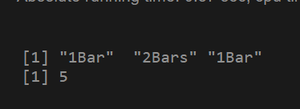Test Case 2: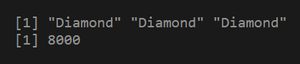Test case 3: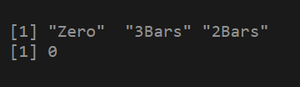Program 2: In this code, we are not passing probabilities parameters.

## R

 `gsy <- ``function``() { ` `    ``s <- ``c``(``"Diamond"``,``"Seven"``,``"3Bars"``,``"2Bars"``,``"1Bar"``,``"Cherry"``,``"Zero"``) ` `    ``sample``(s,size=3,replace=``TRUE``) ` `} ` ` `  `gs<- ``function``(s) { ` `  ``# checking for same symbols ` `   ``s1<- s == s && s == s  ` `  ``b <- s %``in``% ``c``(``"1Bar"``,``"2Bars"``,``"3bars"``) ` `  ``if ``(s1) { ` `    ``cash <- ``c``(``"Diamond"``=1000,``"7"``=800,``"3Bars"``=600,``"2Bars"``=400, ` `              ``"1Bar"``=250,``"Cherry"``=100,``"Zero"``=0) ` `    ``cash_p <- ``unname``(cash[s])  ` `  ``}  ` `   `  `  ``# checking for all bars ` `  ``else` `if ``(``all``(b)) {  ` `    ``cash_p <- 5 ` `  ``} ``else` `{ ` `    ``# checking for cherries ` `    ``cherry1 <- ``sum``(s == ``"Cherry"``)  ` `    ``cash_p <- ``c``(0,2,5)[cherry1+1] ` `  ``} ` `   `  `# checking for diamonds ` `d1 <- ``sum``(s == ``"Diamond"``)  ` `cash_p * 2 ^ d1 ` `} ` ` `  ` `  `run <- ``function``() {  ` `    ``s <- ``gsy``() ` `    ``print``(s) ` `    ``gs``(s) ` `} ` `run``()`

Output:

Test case 1: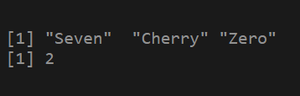Test case 2: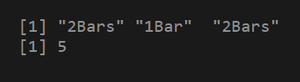Test case 3: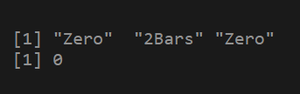Whether you're preparing for your first job interview or aiming to upskill in this ever-evolving tech landscape, GeeksforGeeks Courses are your key to success. We provide top-quality content at affordable prices, all geared towards accelerating your growth in a time-bound manner. Join the millions we've already empowered, and we're here to do the same for you. Don't miss out - check it out now!

Previous
Next